A parallel plate capacitor without any dielectric within its plates, has a capacitance C, and is connected to a battery of emf V. The battery is disconnected and the plates of the capacitor are pulled apart until the separation between the plates is doubled. What is the work done by the agent pulling the plates apart, in this process?
• a)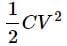• b)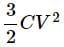• c)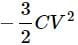• d)
CV2

### Related TestStepway Academy Feb 11, 2022Capacitance of a parallel plate capacitor is C = ε0A/d ……(i)
where A is the area of each plate and d is the distance between the plates. Initial energy stored in the capacitor,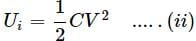When the separation between the plates is doubled , its capcaitance becomes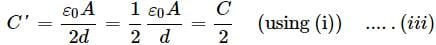As the bettary is disconnected , so charged capacitor becomes isolated and charge on it will reamain constant ,
∴ Q = Q
C'V = C'V (AsQ = CV)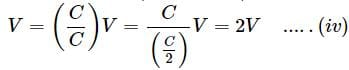Final energy stored in the capacitor ,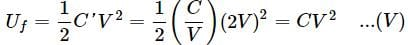Required work done,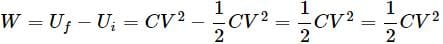Capacitance of a parallel plate capacitor is C = ε0A/d ……(i)where A is the area of each plate and d is the distance between the plates. Initial energy stored in the capacitor,When the separation between the plates is doubled , its capcaitance becomesAs the bettary is disconnected , so charged capacitor becomes isolated and charge on it will reamain constant ,∴ Q = QCV =CV (AsQ = CV)Final energy stored in the capacitor ,Required work done,
Do you know? How Toppers prepare for NEET Exam
With help of the best NEET teachers & toppers, We have prepared a guide for student who are preparing for NEET : 15 Steps to clear NEET Exam